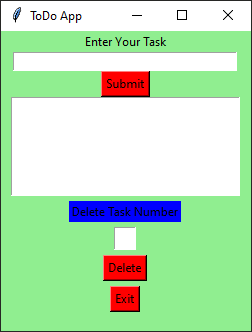# Python | ToDo GUI Application using Tkinter

• Last Updated : 10 Jun, 2021

Prerequisites : Introduction to tkinter

Python offers multiple options for developing GUI (Graphical User Interface). Out of all the GUI methods, Tkinter is the most commonly used method. In this article, we will learn how to create a ToDo GUI application using Tkinter, with a step-by-step guide.

To create a tkinter :

• Importing the module – tkinter
• Create the main window (container)
• Add any number of widgets to the main window.
• Apply the event Trigger on the widgets.

The GUI would look like below:Let’s create a GUI based simple ToDo application in which you can add and delete the Task.

Below is the implementation :

## Python3

 `# import all functions from the tkinter   ` `from` `tkinter ``import` `*`   `# import messagebox class from tkinter` `from` `tkinter ``import` `messagebox`   `# global list is declare for storing all the task` `tasks_list ``=` `[]`   `# global variable is declare for counting the task` `counter ``=` `1`   `# Function for checking input error when` `# empty input is given in task field` `def` `inputError() :` `    `  `    ``# check for enter task field is empty or not` `    ``if` `enterTaskField.get() ``=``=` `"" :` `        `  `        ``# show the error message` `        ``messagebox.showerror(``"Input Error"``)` `        `  `        ``return` `0` `    `  `    ``return` `1`   `# Function for clearing the contents` `# of task number text field` `def` `clear_taskNumberField() :` `    `  `    ``# clear the content of task number text field` `    ``taskNumberField.delete(``0.0``, END)`   `# Function for clearing the contents` `# of task entry field   ` `def` `clear_taskField() :`   `    ``# clear the content of task field entry box` `    ``enterTaskField.delete(``0``, END)` `    `  `# Function for inserting the contents` `# from the task entry field to the text area ` `def` `insertTask():`   `    ``global` `counter` `    `  `    ``# check for error` `    ``value ``=` `inputError()`   `    ``# if error occur then return` `    ``if` `value ``=``=` `0` `:` `        ``return`   `    ``# get the task string concatenating` `    ``# with new line character` `    ``content ``=` `enterTaskField.get() ``+` `"\n"`   `    ``# store task in the list` `    ``tasks_list.append(content)`   `    ``# insert content of task entry field to the text area` `    ``# add task one by one in below one by one` `    ``TextArea.insert(``'end -1 chars'``, ``"[ "` `+` `str``(counter) ``+` `" ] "` `+` `content)`   `    ``# incremented` `    ``counter ``+``=` `1`   `    ``# function calling for deleting the content of task field` `    ``clear_taskField()`   `# function for deleting the specified task` `def` `delete() :` `    `  `    ``global` `counter` `    `  `    ``# handling the empty task error` `    ``if` `len``(tasks_list) ``=``=` `0` `:` `        ``messagebox.showerror(``"No task"``)` `        ``return`   `    ``# get the task number, which is required to delete` `    ``number ``=` `taskNumberField.get(``1.0``, END)`   `    ``# checking for input error when` `    ``# empty input in task number field` `    ``if` `number ``=``=` `"\n"` `:` `        ``messagebox.showerror(``"input error"``)` `        ``return` `    `  `    ``else` `:` `        ``task_no ``=` `int``(number)`   `    ``# function calling for deleting the` `    ``# content of task number field` `    ``clear_taskNumberField()` `    `  `    ``# deleted specified task from the list` `    ``tasks_list.pop(task_no ``-` `1``)`   `    ``# decremented ` `    ``counter ``-``=` `1` `    `  `    ``# whole content of text area widget is deleted` `    ``TextArea.delete(``1.0``, END)`   `    ``# rewriting the task after deleting one task at a time` `    ``for` `i ``in` `range``(``len``(tasks_list)) :` `        ``TextArea.insert(``'end -1 chars'``, ``"[ "` `+` `str``(i ``+` `1``) ``+` `" ] "` `+` `tasks_list[i])` `    `    `# Driver code ` `if` `__name__ ``=``=` `"__main__"` `:`   `    ``# create a GUI window` `    ``gui ``=` `Tk()`   `    ``# set the background colour of GUI window ` `    ``gui.configure(background ``=` `"light green"``)`   `    ``# set the title of GUI window` `    ``gui.title(``"ToDo App"``)`   `    ``# set the configuration of GUI window ` `    ``gui.geometry(``"250x300"``)`   `    ``# create a label : Enter Your Task` `    ``enterTask ``=` `Label(gui, text ``=` `"Enter Your Task"``, bg ``=` `"light green"``)`   `    ``# create a text entry box ` `    ``# for typing the task` `    ``enterTaskField ``=` `Entry(gui)`   `    ``# create a Submit Button and place into the root window` `    ``# when user press the button, the command or ` `    ``# function affiliated to that button is executed ` `    ``Submit ``=` `Button(gui, text ``=` `"Submit"``, fg ``=` `"Black"``, bg ``=` `"Red"``, command ``=` `insertTask)`   `    ``# create a text area for the root` `    ``# with lunida 13 font` `    ``# text area is for writing the content` `    ``TextArea ``=` `Text(gui, height ``=` `5``, width ``=` `25``, font ``=` `"lucida 13"``)`   `    ``# create a label : Delete Task Number` `    ``taskNumber ``=` `Label(gui, text ``=` `"Delete Task Number"``, bg ``=` `"blue"``)` `                       `  `    ``taskNumberField ``=` `Text(gui, height ``=` `1``, width ``=` `2``, font ``=` `"lucida 13"``)`   `    ``# create a Delete Button and place into the root window` `    ``# when user press the button, the command or ` `    ``# function affiliated to that button is executed .` `    ``delete ``=` `Button(gui, text ``=` `"Delete"``, fg ``=` `"Black"``, bg ``=` `"Red"``, command ``=` `delete)`   `    ``# create a Exit Button and place into the root window` `    ``# when user press the button, the command or ` `    ``# function affiliated to that button is executed .` `    ``Exit ``=` `Button(gui, text ``=` `"Exit"``, fg ``=` `"Black"``, bg ``=` `"Red"``, command ``=` `exit)`   `    ``# grid method is used for placing ` `    ``# the widgets at respective positions ` `    ``# in table like structure.` `    ``enterTask.grid(row ``=` `0``, column ``=` `2``)`   `    ``# ipadx attributed set the entry box horizontal size               ` `    ``enterTaskField.grid(row ``=` `1``, column ``=` `2``, ipadx ``=` `50``)` `                       `  `    ``Submit.grid(row ``=` `2``, column ``=` `2``)` `        `  `    ``# padx attributed provide x-axis margin ` `    ``# from the root window to the widget.` `    ``TextArea.grid(row ``=` `3``, column ``=` `2``, padx ``=` `10``, sticky ``=` `W)` `                       `  `    ``taskNumber.grid(row ``=` `4``, column ``=` `2``, pady ``=` `5``)` `                       `  `    ``taskNumberField.grid(row ``=` `5``, column ``=` `2``)`   `    ``# pady attributed provide y-axis` `    ``# margin from the widget.                  ` `    ``delete.grid(row ``=` `6``, column ``=` `2``, pady ``=` `5``)` `                       `  `    ``Exit.grid(row ``=` `7``, column ``=` `2``)`   `    ``# start the GUI ` `    ``gui.mainloop()`

Output:

My Personal Notes arrow_drop_up
Recommended Articles
Page :